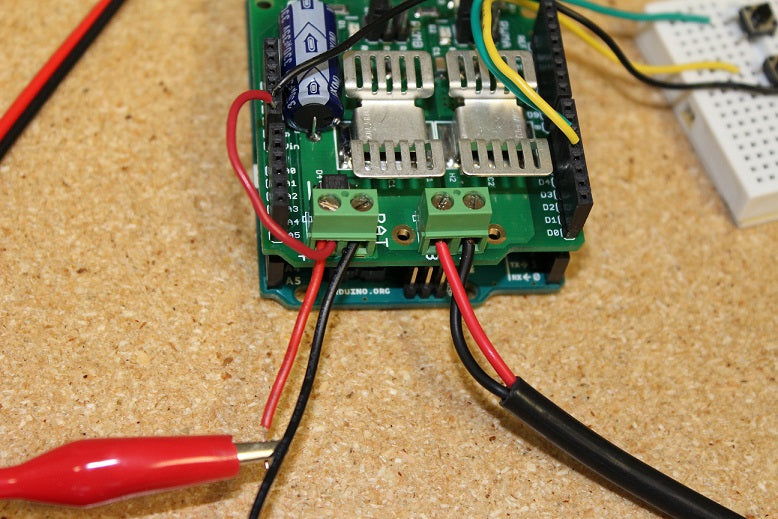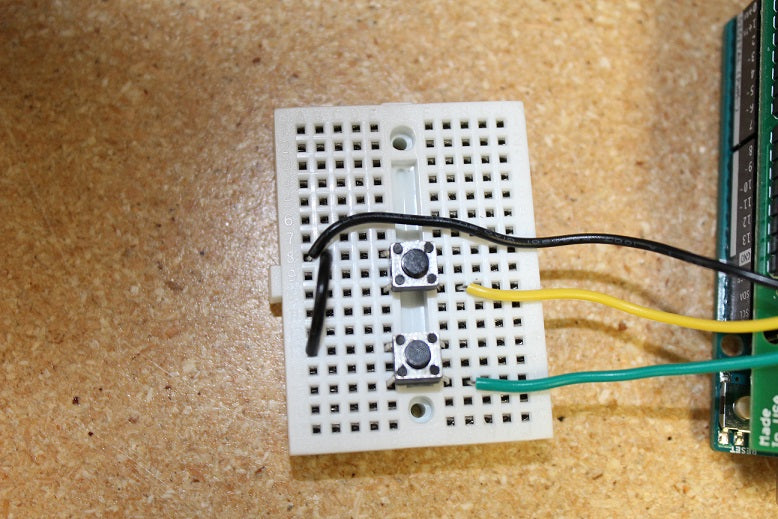# How To Monitor Feedback Of A Linear Actuator Part 1PA Engineer

In today’s guide we will be going over how to find out how much force a linear actuator is applying by monitoring the amount of current it is using. This will be one of our more advanced guides and will require some complex coding, calibrating and programming. We will be covering the monitoring of an analog input and how it utilizes its functions. For this project we’ll be using a MegaMoto Plus, a linear actuator (we are using our PA-14 mini actuator), an Arduino Uno and a power supply of at least 12V.To start off we’ll need to do wiring to connect everything together. Start by plugging the MegaMoto into the Arduino, just place the MegaMoto on top of the Uno to do so. Then connect a wire from the BAT+ terminal found on the MegaMoto to the Vin pin found on the Uno.Now we need to connect the linear actuators wires to the A and B terminals on the MegaMoto and connect the 12V power source to BAT+ and GND to BAT-. We’ll also need to wire two buttons for control by wiring them each between an unused pin and GND. We recommend wiring the buttons on to a breadboard.Now it is time for some coding with the Arduino Uno. We want to program the buttons so that they can control when the actuator will extend and retract. The current will start to be monitored once the actuator extends and this will let us observe whether it surpasses the max current limit or not. If it does pass the limit then the actuator will automatically stop until you choose to retract it. Taking into account that the motors inside actuators have a large current spike when they are first powered, the code we enter will have a short delay before it starts monitoring the current. This code will be able to read when the actuator has reached its limit switches, which is when the current drops to 0.

```const int EnablePin = 8;
const int PWMPinA = 11;
const int PWMPinB = 3; // pins for Megamoto

const int buttonLeft = 4;
const int buttonRight = 5;//buttons to move the motor

const int CPin1 = A5;  // motor feedback

int leftlatch = LOW;
int rightlatch = LOW;//motor latches (used for code logic)

int hitLimits = 0;//start at 0
int hitLimitsmax = 10;//values to know if travel limits were reached

long lastfeedbacktime = 0; // must be long, else it overflows
int firstfeedbacktimedelay = 750; //first delay to ignore current spike
int feedbacktimedelay = 50; //delay between feedback cycles, how often you want the motor to be checked
long currentTimefeedback = 0; // must be long, else it overflows

int debounceTime = 300; //amount to debounce buttons, lower values makes the buttons more sensitive
long lastButtonpress = 0; // timer for debouncing
long currentTimedebounce = 0;

int CRaw = 0;      // input value for current readings
int maxAmps = 0; // trip limit

bool dontExtend = false;
bool firstRun = true;
bool fullyRetracted = false;//program logic

void setup()
{
Serial.begin(9600);
pinMode(EnablePin, OUTPUT);
pinMode(PWMPinA, OUTPUT);
pinMode(PWMPinB, OUTPUT);//Set motor outputs
pinMode(buttonLeft, INPUT);
pinMode(buttonRight, INPUT);//buttons

digitalWrite(buttonLeft, HIGH);
digitalWrite(buttonRight, HIGH);//enable internal pullups
pinMode(CPin1, INPUT);//set feedback input

currentTimedebounce = millis();
currentTimefeedback = 0;//Set initial times

maxAmps = 15;// SET MAX CURRENT HERE

}//end setup

void loop()
{
latchButtons();//check buttons, see if we need to move

moveMotor();//check latches, move motor in or out

}//end main loop

void latchButtons()
{
{
currentTimedebounce = millis() - lastButtonpress;// check time since last press
if (currentTimedebounce > debounceTime && dontExtend == false)//once you've tripped dontExtend, ignore all forwards presses
{
leftlatch = !leftlatch;// if motor is moving, stop, if stopped, start moving
firstRun = true;// set firstRun flag to ignore current spike
fullyRetracted = false; // once you move forwards, you are not fully retracted
lastButtonpress = millis();//store time of last button press
return;
}//end if
}//end btnLEFT

{
currentTimedebounce = millis() - lastButtonpress;// check time since last press

if (currentTimedebounce > debounceTime)
{
rightlatch = !rightlatch;// if motor is moving, stop, if stopped, start moving
firstRun = true;// set firstRun flag to ignore current spike
lastButtonpress = millis();//store time of last button press
return;    }//end if
}//end btnRIGHT
}//end latchButtons

void moveMotor()
{
if (leftlatch == HIGH) motorForward(255); //speed = 0-255
if (leftlatch == LOW) motorStop();
if (rightlatch == HIGH) motorBack(255); //speed = 0-255
if (rightlatch == LOW) motorStop();

}//end moveMotor

void motorForward(int speeed)
{
while (dontExtend == false && leftlatch == HIGH)
{
digitalWrite(EnablePin, HIGH);
analogWrite(PWMPinA, speeed);
analogWrite(PWMPinB, 0);//move motor
if (firstRun == true) delay(firstfeedbacktimedelay); // bigger delay to ignore current spike
else delay(feedbacktimedelay); //small delay to get to speed

getFeedback();
firstRun = false;

latchButtons();//check buttons again
}//end while

}//end motorForward

void motorBack (int speeed)
{
while (rightlatch == HIGH)
{
digitalWrite(EnablePin, HIGH);
analogWrite(PWMPinA, 0);
analogWrite(PWMPinB, speeed);//move motor
if (firstRun == true) delay(firstfeedbacktimedelay);// bigger delay to ignore current spike
else delay(feedbacktimedelay); //small delay to get to speed
getFeedback();

firstRun = false;

latchButtons();//check buttons again

}//end while

dontExtend = false;//allow motor to extend again, after it has been retracted

}//end motorBack

void motorStop()
{
analogWrite(PWMPinA, 0);
analogWrite(PWMPinB, 0);

digitalWrite(EnablePin, LOW);
firstRun = true;//once the motor has stopped, reenable firstRun to account for startup current spikes

}//end stopMotor

void getFeedback()
{

if (CRaw == 0 && hitLimits < hitLimitsmax) hitLimits = hitLimits + 1;
else hitLimits = 0; // check to see if the motor is at the limits and the current has stopped

if (hitLimits == hitLimitsmax && rightlatch == HIGH)
{
rightlatch = LOW; // stop motor
fullyRetracted = true;
}//end if

else if (hitLimits == hitLimitsmax && leftlatch == HIGH)
{
leftlatch = LOW;//stop motor
hitLimits = 0;
}//end if

if (CRaw > maxAmps)
{
dontExtend = true;
leftlatch = LOW; //stop if feedback is over maximum
}//end if

lastfeedbacktime = millis();//store previous time for receiving feedback
}//end getFeedback```